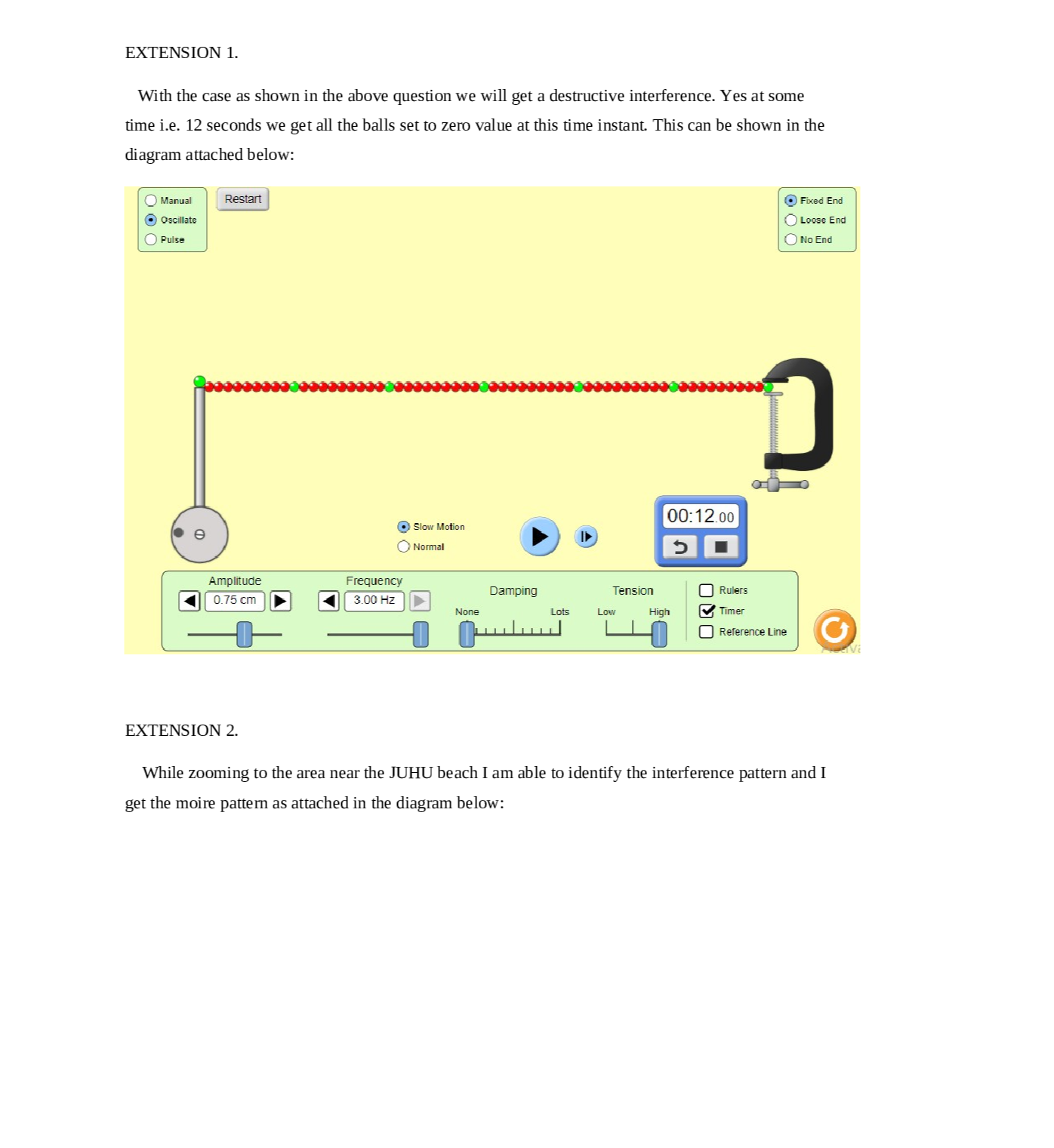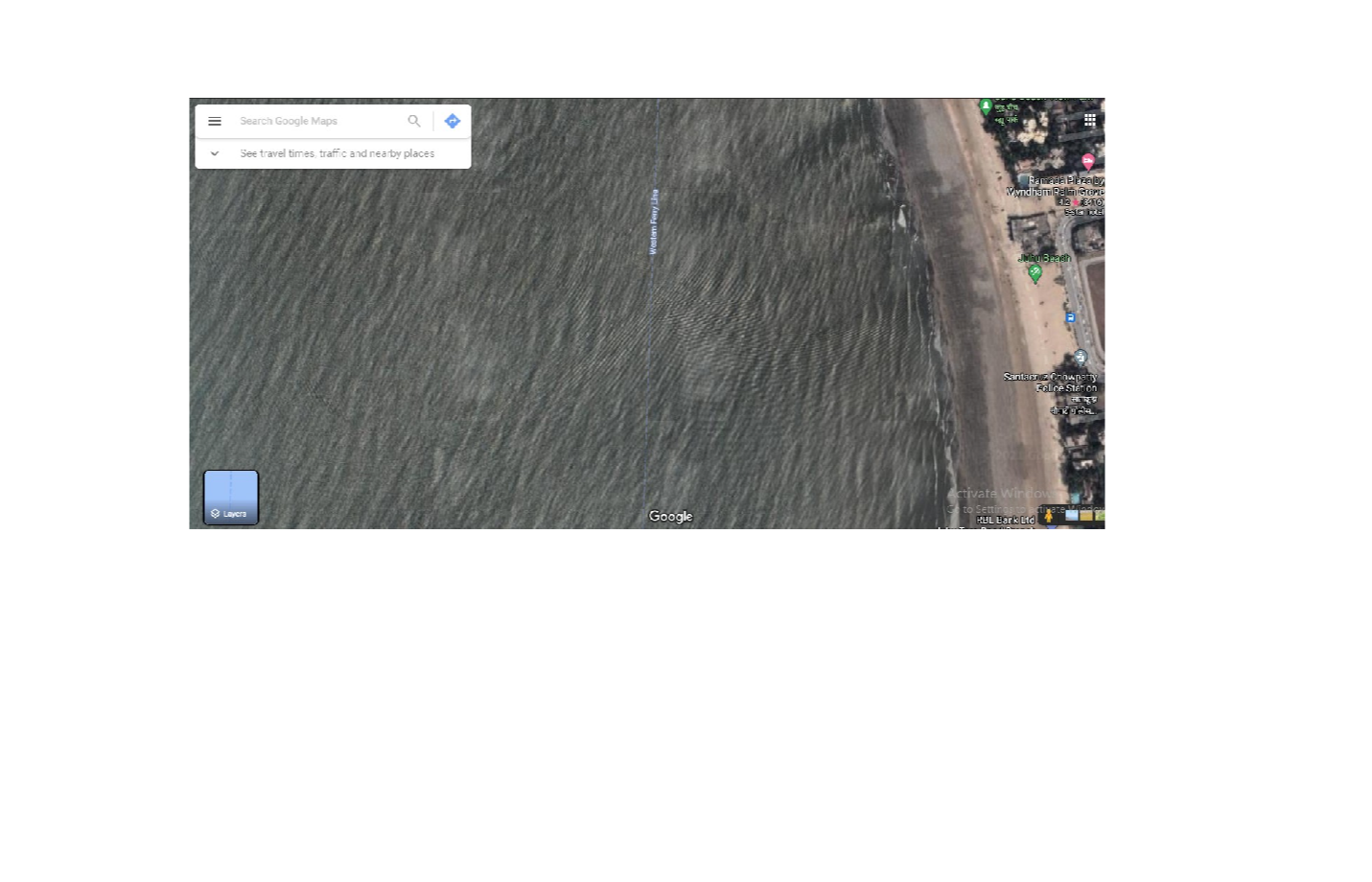Question

Sound and Waves

12. Sometimes in the ocean a "rogue wave" will seemingly come out of nowhere, tower over small ships and quickly disappear. They can be quite dangerous. From what you have done, explain in a sentence or two what might cause rogue waves.

Extension 1: Set it on oscillate, high tension, no damping, slow motion and set the frequency to3 Hz. Will it make destructive interference? Constructive interference? Will the destructive ever reset allI balls to zero at the same time? Hint – let it run through at least 33 cycles. There isa marker on the camshaft that you can use to count them.

Extension 3: (Fun extra example, not graded) Moire patterns using two combs.

Extension 4: (Fun extra example, not graded) Read about "Gravity Waves" which have several meanings. One is a kind of cloud produced from an atmospheric disturbance. They can propagate for hundreds of miles. Be sure to search for "Gravity Wave Images" so you can start watching for them in the sky. The second kind of gravity waves has to do with large interacting galactic bodies and are very strange and complex.

Extension 2: Below is a Moire pattern. These patterns result from constructive and destructive interference between two different sources. Use your favorite mapping application, set it to satellite images, and navigate around a beach of your choice. Zoom in close enough to see the waves. Can you find interference patterns? You might have to look around some points, hooks-or even small islands. Attach a photo showing the interference pattern below.Verified### Question 45059Sound and Waves

A. What is the half-life of 20 g of a radioactive sample if 5 g remain after 8 minutes? (1 point)
B. Assume you have a 500 g sample of a radioactive isotope with a half-life of 100 years. Fillin the table showing how many grams of material will remain after the indicated time has passed. (2 points)
C. How is the half-life of a radioactive material useful for radioactive dating? (1 point)

### Question 45058Sound and Waves

Use the periodic table to answer the following questions.
A. What kind of radioactive decay does not cause transmutation? (1 point)
B. The radioactive atom 210/84PO decays by alpha emission. Write an decay.equation showing the decay
C. The radioactive atom 61270 emits a beta particle. Write an point)equation showing the decay. (1
\text { D. Use the nuclear decay reaction }{ }_{90}^{234} \mathrm{Th} \rightarrow{ }_{91}^{234} \mathrm{~Pa}+{ }_{-1}^{0} \mathrm{e} \text { to answer the following questions. (2 }
i. How does the reaction demonstrate the laws of conservation of charge and conservation of nucleon number? (1 point)
(1ii. What is the binding energy of one mole of 23490 th if the mass defect is 10908 g/mol

### Question 45057Sound and Waves

A. Describe the role of each of the following particles in the structures of protons andneutrons. (2 points)
i. Quarks (1 point)
ii. Gluons (1 point)
B. What forces are at work inside the nucleus of an atom? What keeps the nucleus together?
C. What makes a nucleus unstable? Which atoms tend to be unstable? (1 point)
D. What is lost in an atom as a result of radioactive decay? What equation relates this loss to energy produced? (1 point)

### Question 45056Sound and Waves

A. What causes specific lines to appear in a line spectrum? (1 point)
B. Match the following terms with their definitions. (2 points)
A. Prism
B. Absorption spectrum
C. Emission spectrum
D. Flame test
E. Spectrum
F. Line spectrum
The frequencies of energy absorbed by an element
A continuous range of wavelengths or frequencies
The specific frequencies of energy transitions in a substance
A material that splits light into its frequencies
Heating a sample to identify it by its spectrum
The frequencies of photons a sample emits

### Question 45055Sound and Waves

A. What do the four quantum numbers describe about an electron? (1 point)
B. Match each quantum number with what it defines about an electron's position or energy.
A. The first quantum number, n
B. The second quantum number, /
C. The third quantum number, m,
D. The fourth quantum number, m,
Defines the electron's orbital
Defines the electron's energy level
Defines the electron's spin
Defines the electron's sublevel
C. Identify a set of four possible quantum numbers for the circled electron in manganese(Mn). (There is more than one correct answer.) (1 point)

### Question 45054Sound and Waves

A. Match the term with its definition. (1 point)
A. Frequency
The direction and speed at which a wave is traveling
B. Wavelength
The distance between two adjacent wave peaks
C. Velocity
The number of waves passing point in 1 second
B. What is the equation that illustrates the relationship between wave velocity, frequency,and wavelength? (1 point)
C. What are the equations that represent how the energy of an electromagnetic wave is related to the frequency of the wave and to the wavelength of the wave? Define h and c in your equations and give their values. (2 points)

### Question 44426Sound and Waves

6) An aircraft is travelling at a Mach number of 0.85 through Earth's atmosphere. If the aircraft is travelling at a speed of 985 km/h, then calculate the temperature of the atmosphere in this area. [A4]

### Question 44425Sound and Waves

5) You attach one side of a 0.61 kg rope to wall and send waves down the rope. If the tension in the rope is 1952 N causing the waves travel through the rope at a speed of 80.0 m/s, then what is the length of the rope? [A 4]

### Question 44424Sound and Waves

4) You are jogging along a road at a speed of 4.50 m/s. A car is driving towards you at a speed of 45.0 m/s and ishonking, You hear the car's honking at a frequency of 660.0 Hz. The speed of so und is 345 m/s.
Draw a picture to help you visual the situation. Label it with important information, and state all of the given values in the question. [C 1]а.
b. What is the name of this phenomenon, and why does it occur? [C 2]
C.What is the frequency that the car is honking at? [A 4]

### Question 44423Sound and Waves

Waves are lapping up on a beach. Two new waves come every 1.6 s and a swimmer measures a distance of 12 m between six troughs. A bottle bobbing up and down in the waves drops 120 cm from the waves' highest point to their lowest point.
Draw a diagram of the waveform, labelling all the information given. [2 C]
What is the amplitude of the wave?
What is the period of the wave?
What is the wavelength of the wave? (show your thinking below)[2T]

### Submit query